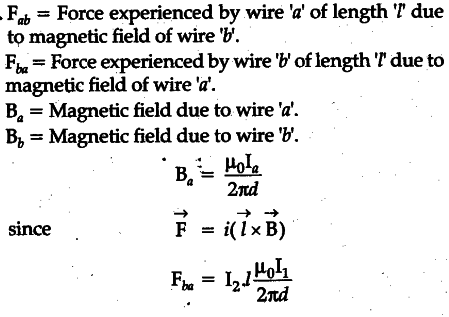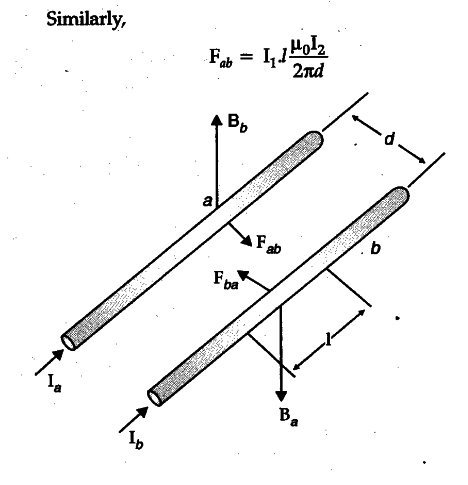# Two long straight parallel conductors carry steady current I1 and I2 separated by a distance d

Two long straight parallel conductors carry steady current I1 and I2 separated by a distance d. If the currents are flowing in file same direction, show how the magnetic field set up in one produces an attractive force on the other. Obtain the expression for this force. Hence define one ampere.The direction of force experienced by the wire ‘a’ is toward the wire ‘b’. (As shown in the diagram).
Similarly the direction of force experienced by the wire ‘b’ is toward the wire Thus, the force is attractive.
If two long wires are placed in vacuum at a separation of 1 m, one Ampere would be defined as the current in each wire that would produce a force of per unit length of wire.